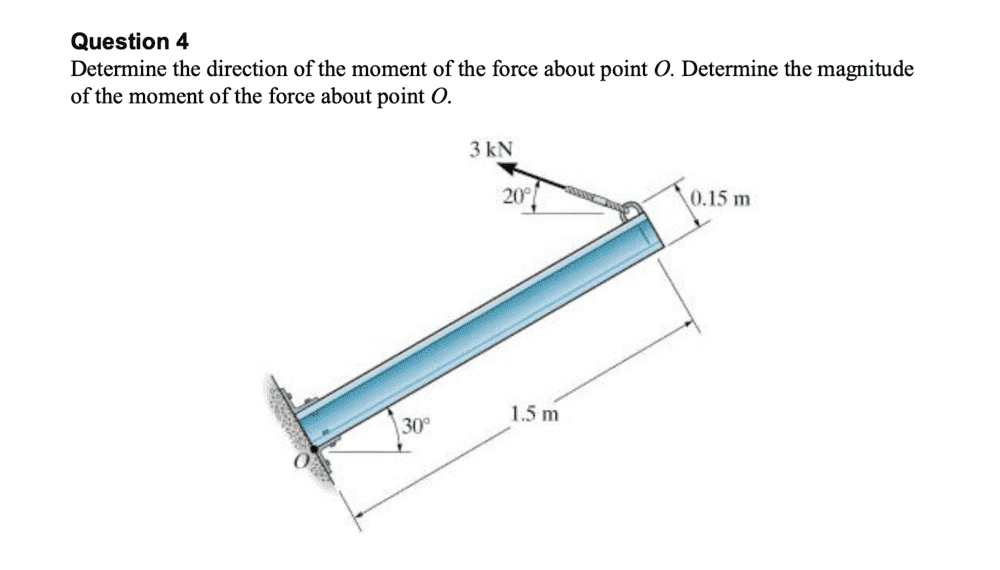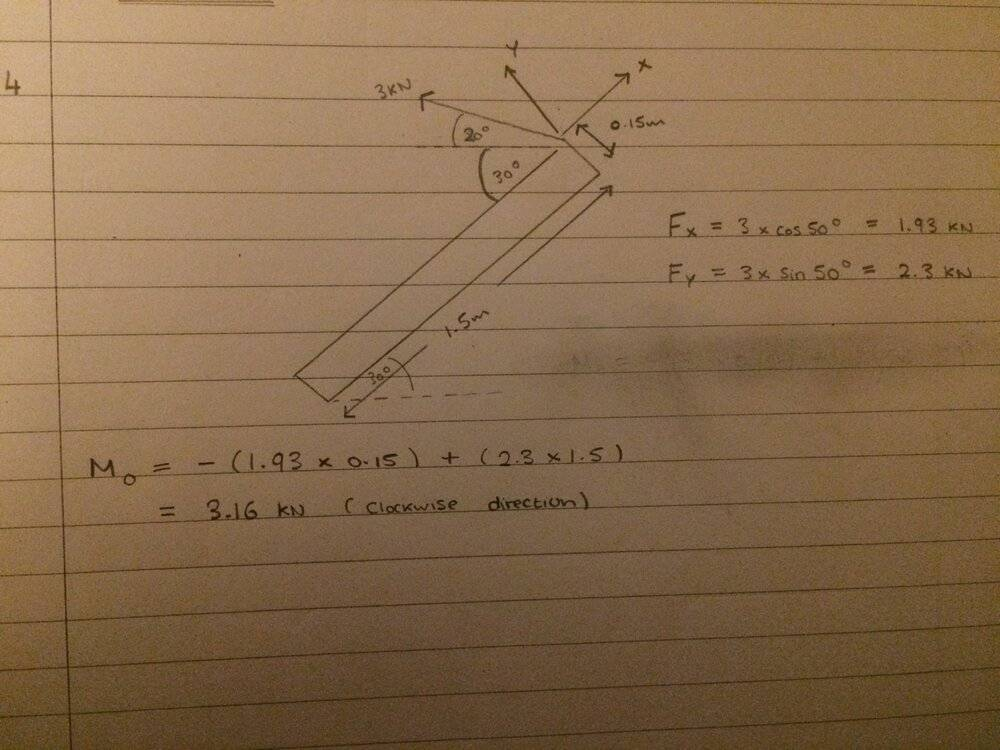# Finding moments about a point

Homework Statement:
See below
Relevant Equations:
moment = force x perpendicular distance
Not sure if I answered this question rightI took my clockwise moments as +ve and counter-clockwise moments as -ve

Any help would be much appreciated! Thanks

TSny
Homework Helper
Gold Member
Should ##F_x## be positive or negative?

Are you sure you took clockwise as positive for the moments? Does ##F_y## tend to rotate the object clockwise or counterclockwise?

•Lnewqban
Should ##F_x## be positive or negative?

Are you sure you took clockwise as positive for the moments? Does ##F_y## tend to rotate the object clockwise or counterclockwise?

Oh it looks like Fy is trying to rotate the bar counter-clockwise
whereas Fx is also rotating it counter-clockwise?

so lets say I took counter-clockwise to be a positive moment, then the moment about O is (Fx * 0.15) + (Fy * 1.5) = 3.74 kN?

•PhanthomJay
Lnewqban
Gold Member
It looks OK to me.

TSny
Homework Helper
Gold Member
Oh it looks like Fy is trying to rotate the bar counter-clockwise
whereas Fx is also rotating it counter-clockwise?
Yes

so lets say I took counter-clockwise to be a positive moment, then the moment about O is (Fx * 0.15) + (Fy * 1.5) = 3.74 kN?
Looks good except for the units of the answer.

•Lnewqban